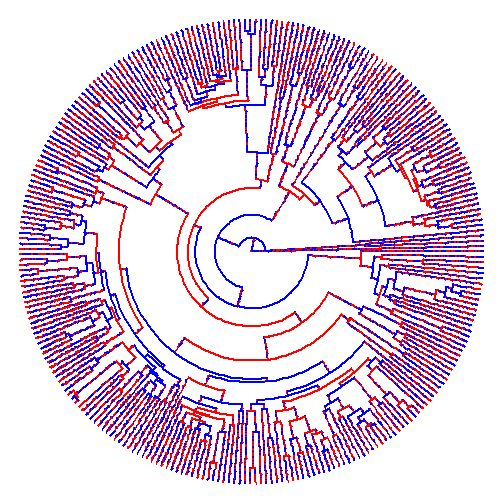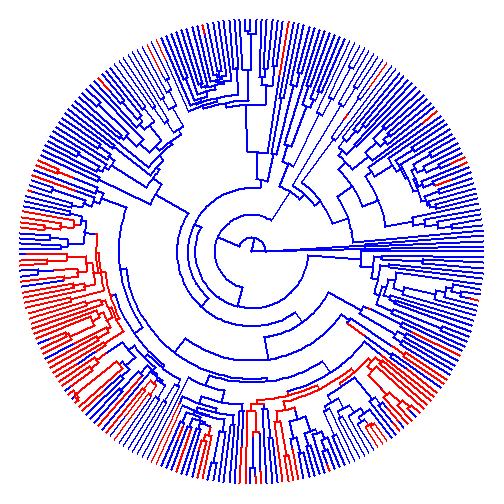## Tuesday, September 20, 2016

### Fix to pass optional `...` arguments to `fitMk` in `make.simmap`

I just pushed a tiny change to `make.simmap` which allows the optional argument `...` to be passed internally to `fitMk` for `Q="empirical"` (i.e., empirical Bayesian, in other words setting Q to its MLE).

The reason for this is that in some circumstances `fitMk`, even though it computes the likelihood no problem, will not converge on the MLE of Q which in turn can cause `make.simmap` to produce a strange result.

Case in point? The example a showed previously in which a failure to find the correct solution for Q causes the number of estimated changes using `make.simmap` to be very large (when it should in fact be quite small).

For instance:

``````library(phytools)
mtree<-make.simmap(tree,x)
``````
``````## make.simmap is sampling character histories conditioned on the transition matrix
##
## Q =
##           0         1
## 0 -1.000294  1.000294
## 1  1.000294 -1.000294
## (estimated using likelihood);
## and (mean) root node prior probabilities
## pi =
##   0   1
## 0.5 0.5
``````
``````## Done.
``````
``````plot(mtree,colors=setNames(c("blue","red"),c(0,1)),
ftype="off",type="fan")
````````````summary(mtree)
``````
``````## 1 tree with a mapped discrete character with states:
##  0, 1
##
## tree has 13731 changes between states
##
## changes are of the following types:
##      0    1
## 0    0 6836
## 1 6895    0
##
## mean total time spent in each state is:
##                 0            1    total
## raw  6873.3187518 6733.8689343 13607.19
## prop    0.5051241    0.4948759     1.00
``````
``````mtree\$logL
``````
``````##  -187.8429
``````

Whereas, when we change the optimizer for Q

``````mtree<-make.simmap(tree,x,opt.method="optim")
``````
``````## make.simmap is sampling character histories conditioned on the transition matrix
##
## Q =
##             0           1
## 0 -0.00510456  0.00510456
## 1  0.00510456 -0.00510456
## (estimated using likelihood);
## and (mean) root node prior probabilities
## pi =
##   0   1
## 0.5 0.5
``````
``````## Done.
``````
``````plot(mtree,colors=setNames(c("blue","red"),c(0,1)),
ftype="off",type="fan")
````````````summary(mtree)
``````
``````## 1 tree with a mapped discrete character with states:
##  0, 1
##
## tree has 71 changes between states
##
## changes are of the following types:
##    0  1
## 0  0 44
## 1 27  0
##
## mean total time spent in each state is:
##                 0            1    total
## raw  1.078702e+04 2820.1642667 13607.19
## prop 7.927445e-01    0.2072555     1.00
``````
``````mtree\$logL
``````
``````##  -155.9019
``````

So far I haven't encountered any problems with this - such as can occur when nested functions use optional arguments differently that happen to have the same name. Please don't hesitate to report any problems.

Good night!